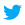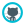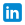# dataewan

## Posterior

The posterior is calculated by taking our pre-observation beliefs, and updating them based on the observed data. The posterior describes the probability distribution of the parameters of the model θ.

The posterior is the object that we do work with. It allows us to make statements about our analysis, and to make predictions about the future.

### Description of the posterior

The posterior distribution is a probability distribution describing the values of the model parameters θ. We want to be able to describe the probability distribution to people, so that they can understand what our model tells them.

One way to describe the distribution is to make point parameter estimates. There are three that you read about a lot.

• The posterior mean
• The posterior median
• The maximum a posteriori (MAP) estimator

The first two are just standard summary statistics of a distibution. The MAP estimator is just a fancy name for the highest point of the probability distribution.

These are easy to report, they give you an estimate of the central point of the probability distribution, which might indicate a likely value for the parameters of your model. There are a couple of problems with a point estimate. One is that each of these estimators will give different results, particularly if there are outliers or the distribution is skewed. The other thing is that the point estimators don't tell you anything about your confidence (or lack of) in your estimates.

Another approach is to report the credible interval of your probability distribution. You might make a report like the 95% credible interval of θ is 0.1 ≤ θ ≤ 0.15, which means that there is a 95% probability that θ is between 0.1 and 0.15. This is straightforward to understand, while giving a way of expressing both the value and the uncertainty in it.

The one caveat with this is that there are usually a large number of regions that describe any credible interval. There are a couple of standard choices you can make to remove this problem. You can choose to report the central posterior interval, which is based on percentiles. You could also report the highest density interval, which just takes the highest parts of the probability distribution. For a symmetrical, unimodal distribution the two intervals will be the same.

### Simulation by sampling from distribution

We can also make predictions from our parameter estimates and our model. We do this by taking samples from our posterior distribution like this:

1. For each of our parameters θ, take a sample value from the posterior distribution
2. Put those parameters back into the model to get a simulated output from the model
3. Repeat this quite a few times, so that you can see the range of values that you're predictingPredictions made by taking samples from a multivariate gaussian model